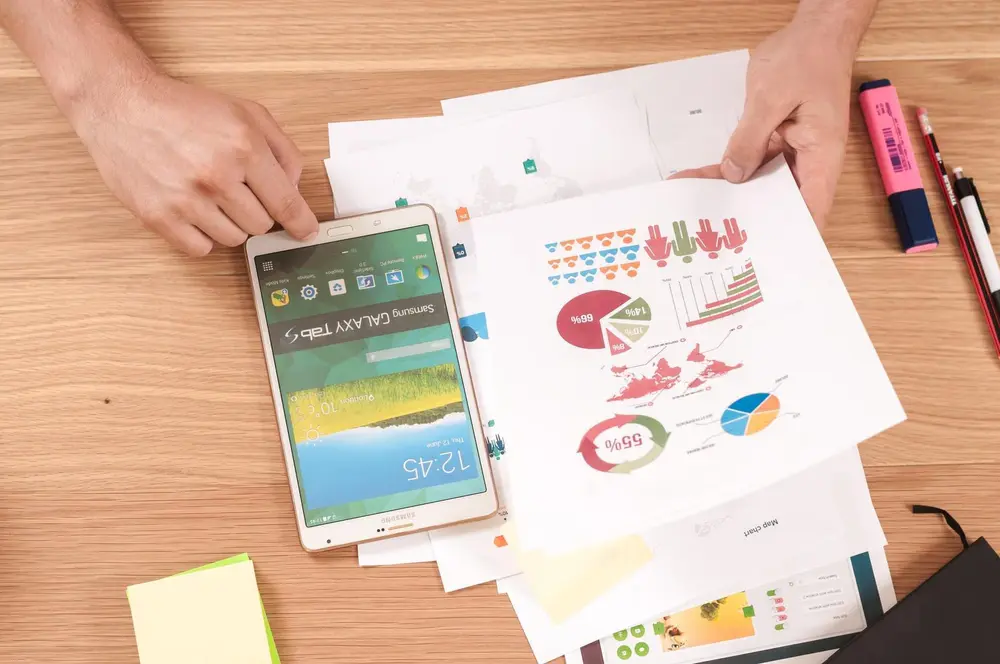# Probability

Probability is how likely an event is to happen. The probability of an event occurring is given as a fraction, decimal or percentage. This is because a probability is only from 0 to 1 inclusive. This is because:

A probability of 0 means the event is NOT going to occur

A probability of 1 means the event is DEFINITELY going to occur

A probability is worked out by finding:

Number of ways the event occurs / Total number of events possible

For example:

What is the probability of throwing a 6 on a dice.
There is 1 possible chance of throwing a six.
There are 6 different out comes possible when throwing a dice.
The probability of throwing a six = 1 / 6

Combining Probabilities
When finding the probability of two or more events occurring one after the other, you need to find out the probability of each of the events occurring. Then you see whether you add or multiply the probabilities.

If one AND another event has to occur you MULTIPLY

If one OR another event has to occur you ADD

You should also know how to illustrate probabilities with possibility space diagrams and tree diagrams.

Handling data

Handling data